# NEET (UG) – 2013 Chemistry Previous Year

Q.46 The value of Planck’s constant is 6.63 × 10–34 Js. The speed of light is 3 × 1017 nm s–1. Which value is closest to the wavelength in nanometer of a quantum of light with frequency of 6 × 1015 s–1 ?

(1) 10             (2) 25          (3) 50          (4) 75

Chemistry Notes

Physics Notes

Biology Notes

Q.47 What is the maximum numbers of electrons that can be associated with the following set of quantum numbers ?

n = 3, l = 1 and m = –1.

(1) 10          (2) 6            (3) 4            (4) 2

Q.49 A hydrogen gas electrode is made by dipping platinum wire in a solution of HCl of pH = 10 and by passing hydrogen gas around the platinum wire at one atm pressure. The oxidation potential of electrode would be ?

(1) 0.059 V                      (2) 0.59 V

(3) 0.118 V                      (4) 1.18 V

Chemistry Notes

Physics Notes

Biology Notes

Q.50 A reaction having equal energies of activation for forward and reverse reactions has –

(1) ΔS = 0                        (2) ΔG = 0

(3) ΔH = 0                       (4) ΔH = ΔG = ΔS = 0

Q.51 At 25ºC molar conductance of 0.1 molar aqueous solution of ammonium hydroxide is 9.54 ohm–1 cm2 mol–1 and at infinite dilution its molar conductance is 238 ohm–1 cm2 mol–1. The degree of ionisation of ammonium hydroxide at the same concentration and temperature is –

(1) 2.080 %                     (2) 20.800 %

(3) 4.008 %                     (4) 40.800 %

Q.52 Based on equation E = –2.178 × 10–18 J(Z2 / n2) , certain conclusions are written. Which of them is not correct ?

(1) The negative sign in equation simply means that the energy of electron bound to the nucleus is lower than it would be if the electrons were at the infinite distance from the nucleus.

(2) Larger the value of n, the larger is the orbit radius.

(3) Equation can be used to calculate the change in energy when the electron changes orbit.

(4) For n = 1, the electron has a more negative energy that it does for n = 6 which means that the electron is more loosely bound in the smallest allowed orbit.

Q 53.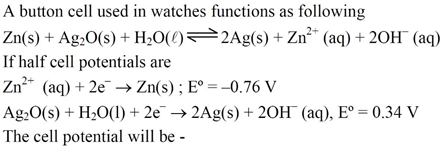(1) 1.10 V              (2) 0.42 V

(3) 0.84 V              (4) 1.34 V

Chemistry Notes

Physics Notes

Biology Notes

Q.54 How many grams of concentrated nitric acid solution should be used to prepare 250 mL of 2.0 M HNO3 ? The concentrated acid is 70 % HNO3.

(1) 45.0 g conc. HNO3              (2) 90.0 g conc. HNO3

(3) 70.0 conc. HNO3                 (4) 54.0 g conc. HNO3

Q.55 The number of carbon atoms per unit cell of diamond unit cell is –

(1) 4     (2) 8     (3) 6      (4) 1

Q.56 Maximum deviation from ideal gas is expected from :

(1) H2(g)                (2) N2(g)

(3) CH4(g)             (4) NH3(g)

Q.57 A metal has a fcc lattice. The edge length of the unit cell is 404 pm. The density of the metal is 2.72 g cm–3. The molar mass of the metal is :

[NA Avogadro’s constant = 6.02 × 1023 mol–1]

(1) 40 g mol–1                            (2) 30 g mol–1

(3) 27 g mol–1                            (4) 20 g mol–1

Chemistry Notes

Physics Notes

Biology Notes

Q.58 Dipole – induced dipole interactions are present in which of the following pairs :

(1) H2O and alcohol                  (2) Cl2 and CCl4

(3) HCl and He atoms                (4) SiF4 and He atoms

Q.59 A magnetic moment of 1.73 BM will be shown by one among the following –

(1) [Cu(NH3)4]2+              (2) [Ni(CN)4]2–

(3) TiCl4                          (4) [CoCl6]4–

Q.60 Roasting of sulphides gives the gas X as a byproduct. This is colorless gas with choking smell of burnt sulphur and causes great damage to respiratory organs as a result of acid rain. Its aqueous solution is acidic, acts as a reducing agent and its acid has never been isolated. The gas X is –

(1) H2S                            (2) SO2

(3) CO2                            (4) SO3

Q.61 Which is the strongest acid in the following ?

(1) H2SO4              (2) HClO3

(3) HClO4              (4) H2SO3

Q.62 Which of the following is paramagnetic ?

(1) CO                             (2) O2

(3) CN                            (4) NO+

Chemistry Notes

Physics Notes

Biology Notes

Q.63 Which of the following structure is similar to graphite ?

(1) BN                             (2) B

(3) B4C                            (4) B2H6

Q.64 The basic structural unit of silicates is –

(1) SiO                           (2) SiO44–

(3) SiO32–                        (4) SiO42–

Q.65 Reaction by which Benzaldehyde cannot be prepared-

(a)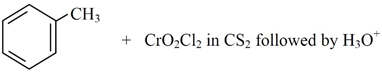(b)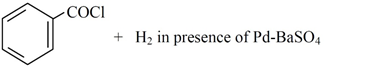(c)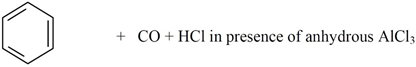(d)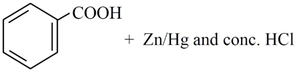Q.66 Which of the following does not give oxygen on heating ?

(1) KClO3                                  (2) Zn(ClO3)2

(3) K2Cr2O7                               (4) (NH4)2Cr2O7

Chemistry Notes

Physics Notes

Biology Notes

Q.67 Which of the following lanthanoid ions is diamagnetic ? (At. No. Ce = 58, Sm = 62, Eu = 63, Yb = 70)

(1) Ce2+                  (2) Sm2+

(3) Eu2+                 (4) Yb2+

Q.68 Identify the correct order of solubility in aqueous medium-

(1) CuS > ZnS > Na2S              (2) ZnS > Na2S > CuS

(3) Na2S > CuS > ZnS              (4) Na2S > ZnS > CuS

Q.69 XeF2 is isostructural with-

(1) TeF2                 (2) ICl2

(3) SbCl3               (4) BaCl2

Q.70 An excess of AgNO3 is added to 100 mL of a 0.01 M solution of dichlorotetraaquachrominum (III) chloride.

The number of moles of AgCl precipitated would be-

(1) 0.001               (2) 0.002

(3) 0.003               (4) 0.01